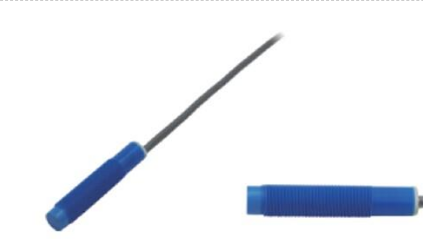# Introduction to five common output sensors

(1) Resistive

This type of sensor converts the measured value into a change in resistance through a sensitive element. For example, platinum resistance and copper resistance temperature sensors convert the measured temperature into changes in resistance value; thermistors also convert the measured temperature into changes in semiconductor resistance; resistance strain sensors measure the load (force) , Weight), torque, torque, tension, tension, speed, acceleration, etc. are converted into resistance value changes of the resistance strain gauge.

The function of the sensor circuit is to convert the change in resistance into electrical parameters that are easy to measure, such as using a bridge to convert the resistance into a voltage or current output; using an oscillation circuit to convert the change in resistance into frequency.(2) Capacitive type

This type of sensor converts the measured component into a change in capacitance through a sensitive element. For example, the capacitive linear displacement and angular displacement sensors change the relative position of the capacitor plates, thereby changing the capacitance; the capacitive liquid level gauge converts the change in liquid level height to the change in capacitance; the capacitive load sensor changes through the deformation of the elastomer The relative position of the capacitor plate changes the capacitance; the capacitive vibration sensor, acceleration sensor, thickness sensor, concentricity sensor, and temperature sensor also convert the measured mechanical vibration, acceleration, thickness, eccentricity, humidity, etc. into capacitance Change accordingly.

The function of the sensor circuit is to convert the change in capacitance into a voltage or current signal that can be easily processed, or into a frequency signal through an oscillating circuit.

(3) Inductive

This type of sensor converts the measured component into a change in inductance through a sensitive element. For example, inductive linear and angular displacement sensors change the relative position of the core with the core's inductance, so that the inductance changes with the measured displacement; the speed and acceleration sensors also change the core's inductance with the speed and acceleration; The pressure sensor is deformed by the elastic element feeling the measured pressure, and the mechanical deformation of the elastic body drives the sensor core displacement to change the inductance.

The function of the sensor circuit is to convert the change in inductance caused by the measured change into a signal form that is easy to process. For example, an inductor bridge is used to convert the change in inductance to a change in current or voltage; an oscillation circuit is used to convert the change in inductance Into frequency changes.

(4) Mutual inductance

This type of sensor converts the measured component into a change in mutual inductance through a sensitive element. Such as a differential transformer type sensor, the measured change is converted into the displacement of the differential transformer core through the transmission of mechanical parts, so that the mutual inductance between the exciting winding and the measurement winding changes; the eddy current sensor is measured by The change is converted into a change in the distance between the measurement coil and the measured object, which causes a change in the mutual inductance and changes in the inductance of the measurement coil.